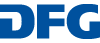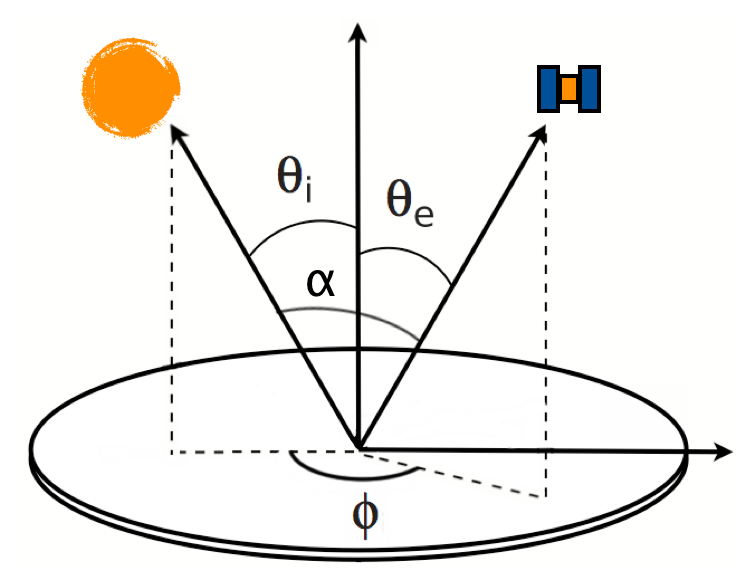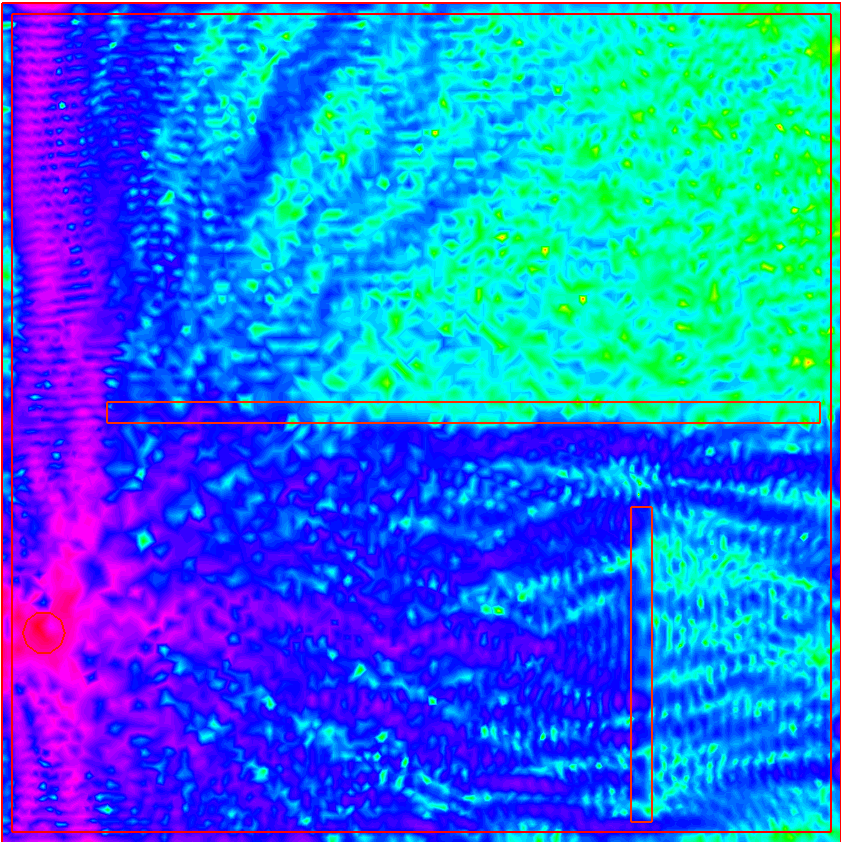You are here:   HomeLight Scattering Software 02 | 07 | 2020
Related / External
Acknowledgement
We acknowledge support of this project by Deutsche Forschungsgemeinschaft (DFG) within the special program "Themenorientierte Informationsnetzwerke".The page is hosted by the University of Bremen.VCRMEll2D

VCRMEll2D by Kuan Fang Renis the first realization of the Vectorial Complex Ray Model - VCRM developed by the author. By introducing the property of the Wavefront in the geometrical optics model, the VCRM can calculate very precisely the interaction of a wave of any form with a object of smooth surface and size much larger than the wavelength of the incident beam.MCML is a steady-state Monte Carlo simulation program for multi-layered turbid media with an infinitely narrow photon beam as the light source. Each layer has its own optical properties of absorption, scattering, anisotropy, and refractive index.

MySimLabs is a web site presenting some Open Source MATLAB tools for simulating how light interacts with opaque surfaces, using Monte Carlo ray-tracing methods by David Bergström.Mie GOMsphere is a geometrical-optics program by Xiaobing Zhou to calculate the scattering coefficients and phase function of unpolarized radiation by large dielectric spheres.

Siris is a ray-optics light-scattering Fortran program by Karri Muinonen.### FreeFEM

FreeFEM is a partial differential equation solver for non-linear multi-physics systems in 2D and 3D. Problems involving partial differential equations from several branches of physics, such as fluid-structure interactions, require interpolations of data on several meshes and their manipulation within one program. FreeFEM includes a fast interpolation algorithm and a language for the manipulation of data on multiple meshes.MATLAB code to compute radar cross section (RCS) of sphere-tipped cone based on Physical-Optic Method by Xiaojian Xu.

### ERMES

ERMES (Electric Regularized Maxwell Equations with Singularities) is a finite element code in frequency domain which implements in C++ a simplified version of the weighted regularized Maxwell equation method.Various ray-tracing codes eg. for light scattering from non-spherical particles containing scattering and absorbing inclusions or from polyhedral shaped crystals by Andreas Macke.

### XLiFE++

XLiFE++ is an FEM-BEM C++ code developed by P.O.e.m.s. laboratory and I.R.M.A.R. laboratory, that can solve 1D / 2D / 3D, scalar / vector, transient / stationnary / harmonic problems.

It includes Boundary Elements methods for Maxwell equations.